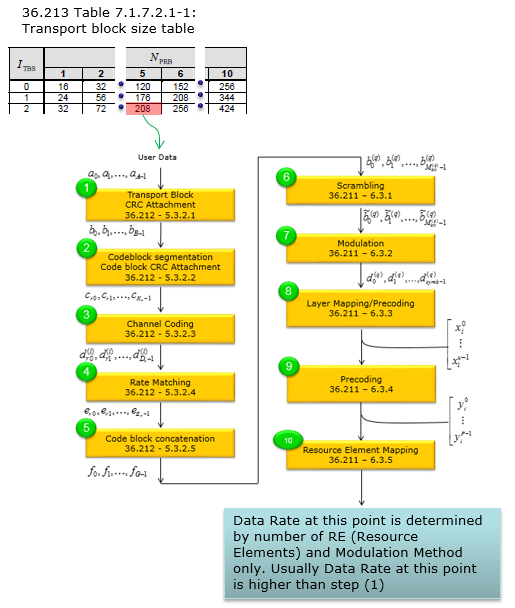LTE Quick Reference Throughput Calculation Example - FDD   If you know the MCS index and number of RBs, you can calculate the throughput for that specific MCS idex and RB as follows:   PHY layer throughput in bits =   Transport Block Size (bits) / subframe                                          x Number of the scheduled subframes / sec                                        =  ???? bits/sec         , where number of transport blocks /subframe is 1 for TM1,TM2 and 2 for TM3, TM4          NOTE 1 : Transport Block Size is determined by the number of RB and MCS according to TS36.213                    Table 7.1.7.1-1 and Table 7.1.7.1-2         NOTE 2 : Number of the scheduled subframe mean that the subframe that is scheduled to transmit data.                       if UE is in max throughput condition, you may assume that every subframe is scheduled to transmit                       user data. In this case, 'Number of the scheduled subframes' become 1000   Calculation Procedure for downlink(PDSCH) is as follows :   i) refer to TS36.213 Table 7.1.7.1-1 ii) get I_TBS for using MCS value (Let's assume MCS is 23. in this case, I_TBS is 21 ) iii) refer to TS36.213 Table7.1.7.2.1 iv) go to column header indicating the number of RB (Let's assume that RB is 100) v) go to row header �21� which is I_TBS vi) you would get 51024 (if the number of RB is 100 and I_TBS is 21) vii) (This is Transfer Block Size per 1 ms for one Antenna)   If we use 2 antenna, the throughput is 51024 bits * 2 transport blocks * 1000 subframes/sec = about 100 Mbps   Calculation Procedure for uplink(PUSCH) is as follows : Same as the downlink as above except that you have to refer to 36.213 Table 8.6.1-1 at step i) Uplink Analysis Paremeter Calculation   Click here for TS 36.213 Tables for TBS     Note :   The throughput calculated in this page is the throughput at the first step of the following process (Refer to Physical Layer Channel : Downlink : PDSCH (Physical Downlink Shared Channel) for the details). Usually throughput calculated at this step is taken as a reference throughput (ideal throughput) because the throughput specified in UE Category represents the throughput at this step. When you say 'Trasport Block Size', it means the size of the array 'a' at the first step of the following process.   This throughput often get configured with the throughput calculated at the last step of this process. The throughput at the last step in the process can be defined as physical layer throughput. The data rate at this step is determined only by number of REs allocated for the data and the modulation method (QPSK, QAM, 16 QAM etc). In most case (practically every PDSCH), there is some difference in terms of data rate between the first step and the last step. This difference is determined by Code Rate.